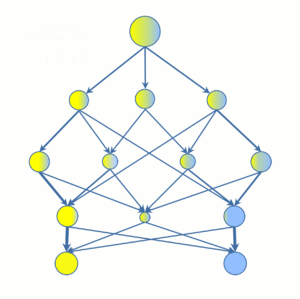# Decision stream graph facts for kids

Kids Encyclopedia FactsFig. 1. Decision stream: statistic-based merge of nodes from the same/different levels of predictive model.

Decision stream is a directed acyclic graph of decision rules for classification and regression tasks (Fig. 1). This decision tree based method avoids the problem of data exhaustion in terminal nodes by merging of leaves from the same/different levels of predictive model.

Decision stream provides:

– High accuracy due to the precise splitting of data with unpaired two-sample test statistics.

– Decrease of overfitting due to partition of data only into statistically representative groups.

– Reduction of complexity on every level of predictive model.

– Self-regulated depth of predictive model.Decision stream graph Facts for Kids. Kiddle Encyclopedia.